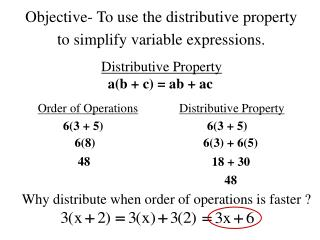Download PresentationObjective- To use the distributive property to simplify variable expressions.

# Objective- To use the distributive property to simplify variable expressions. - PowerPoint PPT PresentationDownload Presentation## Objective- To use the distributive property to simplify variable expressions.

- - - - - - - - - - - - - - - - - - - - - - - - - - - E N D - - - - - - - - - - - - - - - - - - - - - - - - - - -
##### Presentation Transcript

1. Objective- To use the distributive property to simplify variable expressions. Distributive Property a(b + c) = ab + ac Order of Operations Distributive Property 6(3 + 5) 6(3 + 5) 6(3) + 6(5) 6(8) 48 18 + 30 48 Why distribute when order of operations is faster ?

2. Use the distributive property to simplify. 1) 3(x + 7) 6) x(a + m) ax + mx 3x + 21 2) 2(a + 4) 7) 4(3 + r) 2a + 8 12 + 4r 3) 7(8 + m) 8) 2(x + 8) 2x + 16 56 + 7m 4) 3(4 + a) 9) 7(2m + 3y + 4) 12 + 3a 14m + 21y + 28 5) (3 + k)5 10) (6 + 2y + a)3 15 + 5k 18 + 6y + 3a

3. Use the distributive property to simplify. 1) 4(y + 7) 6) a(c + d) 4y + 28 ac + ad 2) 3(b + 6) 7) 8(3 + r) 3b + 18 24 + 8r 3) 5(9 + m) 8) 4(x + y + 1) 45 + 5m 4x + 4y + 4 4) 5(4 + a) 9) 5(2m + 3 + t) 20 + 5a 10m + 15 + 5t 5) (7 + k)6 10) (11 + 2y)+3y 42 + 6k 11 + 5y

4. Geometric Model for Distributive Property 3 7 4 Two ways to find the total area. Width by total length Sum of smaller rectangles 4(3 + 7)

5. Geometric Model for Distributive Property 3 7 4 4(3) 4(7) Two ways to find the total area. Width by total length Sum of smaller rectangles = 4(3 + 7) 4(3) + 4(7)

6. Geometric Model for Distributive Property 4 x 9 Two ways to find the total area. Width by total length Sum of smaller rectangles = 9(4 + x) 9(4) + 9(x)

7. Factoring Find the missing factor. 1) 2) 3) 4) 5)

8. Find the GCF of the following mentally. 1) 2) 3) 4) Factoring Greatest Common Factor GCF -

9. Factor the following expression. 1) 3) 2) 4) Factoring One type involves the Distributive Property in reverse.

10. Factoring One type involves the Distributive Property in reverse. Factor the following expression. 5) 7) 6) 8)

11. Like Terms Like terms - variable terms that differ only in their coefficients. Unlike Terms Like Terms 7x 3y 7x 3x 5y y 5y 10xy 2yx 2a 3ab

12. Simplify by combining like terms. 1) 7x + 4y + 2x + y 5) 4k + (2j) + 6k + j + 3j 10k 9x + 5y 2) 3m + 10n + 4m + 1 6) 10 + 4x + 3y + 17x 21x 7m + 10n + 1 + 3y + 10 7) 3) 7x + 3y 7x + 3y 4) 6x + (5x) + 2x + 3 8) 13x + 3

13. Simplify the expression using the Distributive Property and then combining like terms. 3) 1) 2) 4)

14. Simplify each variable expression. 5) 7(x + 4) + 2x 8) 3(b + 2) + 6b 7x + 28 + 2x 3b + 6 + 6b 9x + 28 9b + 6 6) 5x + 3(x + 1) 9) 2(3m + 1) + 4m 5x + 3x + 3 6m + 2 + 4m 8x + 3 10m + 2 7) a + 2(4 + a ) + 1 10) 3(k + m) + 5(k + 2m) + 5k + 10m a + 8 + 2a + 1 3k + 3m 3a + 9 8k + 13m